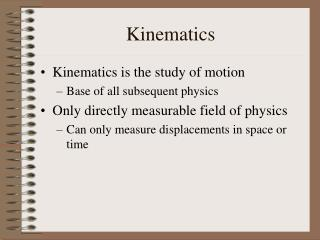DownloadDownload PresentationKinematics

# Kinematics

Télécharger la présentation## Kinematics

- - - - - - - - - - - - - - - - - - - - - - - - - - - E N D - - - - - - - - - - - - - - - - - - - - - - - - - - -
##### Presentation Transcript

1. Kinematics • Kinematics is the study of motion • Base of all subsequent physics • Only directly measurable field of physics • Can only measure displacements in space or time

2. Frames of Reference • In order to quantify any measurement, a Frame of Reference must be defined. • Frames of reference use coordinate axes to quantify measurements • Coordinate axes are just number lines! • Number of orthogonal axes = number of dimensions

3. Definition of Position • Position: Where an object is located. • Usually measured relative to the origin, but not necessary • Includes direction along the axis. • Displacement is how far an object is from a reference point • Includes direction along the axis.

4. Example: Let an object be located at x = 4 m. Relative to the point x0 = 1 m, the displacement isDx = x – x0 = 4 m – 1 m = 3 m.Let another object be located at x’ = -5 m. The displacement to this point isDx’ = x’ – x0 = -5 m – 1 m = -6 m.

5. Definition of Velocity • Velocity: The rate at which the position of an object changes. • Think of as how fast an object is moving. • Includes direction along the axis • Arrow reminds us to think about direction • This definition is the average velocity • Uses ending & starting position and time • Doesn’t take into account what happens in between • Speed: Magnitude of velocity • No directional information

6. Definition of Acceleration • Acceleration: The rate at which the velocity of an object changes • Includes direction along axis • Tells how velocity changes; does not address velocity itself.

7. Equations of motion • Assume initial time is zero, initial position, x0, is position at t = 0, and initial velocity, v0, is velocity at t = 0. • Assume final position and velocity are functions of time, x = x(t) and v = v(t), respectively. • Assume acceleration is constant • Start with definition of acceleration

8. If the velocity is constant (a = 0), then the definition of velocity can be rearranged as • If acceleration is not zero, then v above is average velocity, vave.

9. Know initial and final velocities, so average is • Substitute into previous equation

10. Substituting equation of velocity yields

11. If the time is not known, can use previous two equations to eliminate it • First solve velocity equation for time, t, • Substitute this into position equation

12. Summary of equations